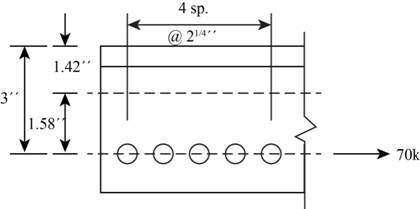Chapter 8, Problem 8.2.4P

Chapter
Section
Textbook Problem

The fasteners in the connection of Figure P8.2-4 are placed at the workable gage distance shown in Manual Table 1-7A. What additional force is experienced as a consequence of the fasteners not being on the centroidal axis of the member?To determine

The additional force experienced as a consequence of the fastener not being on the centroidal axis of the member.

Explanation

Concept used:

Write the expression for total shear force in the fastener.

p=(px2)+(py2)

Here, the total load coming on critical bolt due to eccentricity in x direction is px, the total load coming on critical bolt due to eccentricity in y direction is py, and the total shear force in fastener is p.

Calculation:

Write the expression for the load which is coming on each of the bolt in x-direction.

Pex=Pxn     ...... (I)

Here, the load component in x direction is Px, load which is coming on each of the bolt in x-direction is Pex, and the number of bolt is n

Substitute 70k for Px and 5 for n in Equation (I).

Pex=70k5=14k

Write the expression for the load on each of the bolt in the y-direction.

Pey=Pyn     ...... (II)

Here, the load component in the y direction is Py, load which is coming on each of the bolt in y-direction is Pey and the number of bolt is n.

There is no load acting in the y direction, so Pey is zero.

Write the expression to calculate the location of the centroid with respect to the lower left bolt.

x¯=(n×d)T ..... (III)

Here, the number of bolts is n, the distance of the bolts is d, and the total number of bolts is T.

Substitute the values in Equation (III).

x¯=(9×1)+(6.75×1)+(4.5×1)+[2.25×1]5=4.5in

Write the expression to calculate the location of the centroid with respect to the lower left bolt.

y¯=(n×d)T ..... (IV)

Here, the centroid of y¯ is zero.

Calculate the eccentricity of the load that is the distance from the middle of the bracket connection to the point of load in both x and y directions from the Figure-1 diagram given below.

Figure-(1)

Calculate the distance of the load from the middle of the connection.

ex=4.5in

ey=1.58in

Here, the eccentricity in x direction is ex and the eccentricity in y direction is ey.

Write the expression to calculate horizontal and vertical components of the loads caused due to eccentricity.

Σ(x2+y2)=(2.25in)2×2+(4.5in)2×2=50.63in2

Write the expression for the moment for x-axis about midpoint of the connection between the bolts.

Mx=Px×ey ..... (V)

Here, moment generated is x direction is Mx.

Substitute 70k for Px and 1.58in for ey in Equation (V).

Mx=70k×1.58in=110.6in-k

Write the expression for the moment for y-axis about midpoint of the connection between the bolts.

My=Py×ex ..... (VI)

Here, moment generated is y direction is My.

There is no load acting y direction, so Pey is zero

Still sussing out bartleby?

Check out a sample textbook solution.

See a sample solution

The Solution to Your Study Problems

Bartleby provides explanations to thousands of textbook problems written by our experts, many with advanced degrees!

Get Started

Why do economics and material selection play important roles in the design decision making process?

Engineering Fundamentals: An Introduction to Engineering (MindTap Course List)

Convert 10,000 ft-lbf of energy into BTU, Joules, and kilojoules.

Fundamentals of Chemical Engineering Thermodynamics (MindTap Course List)

What is the basic difference between a Delco ABS VI and other typical systems?

Automotive Technology: A Systems Approach (MindTap Course List)